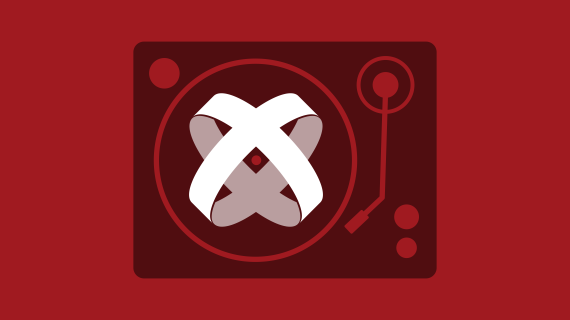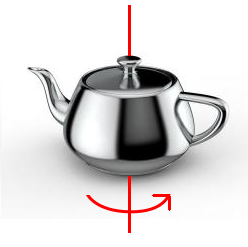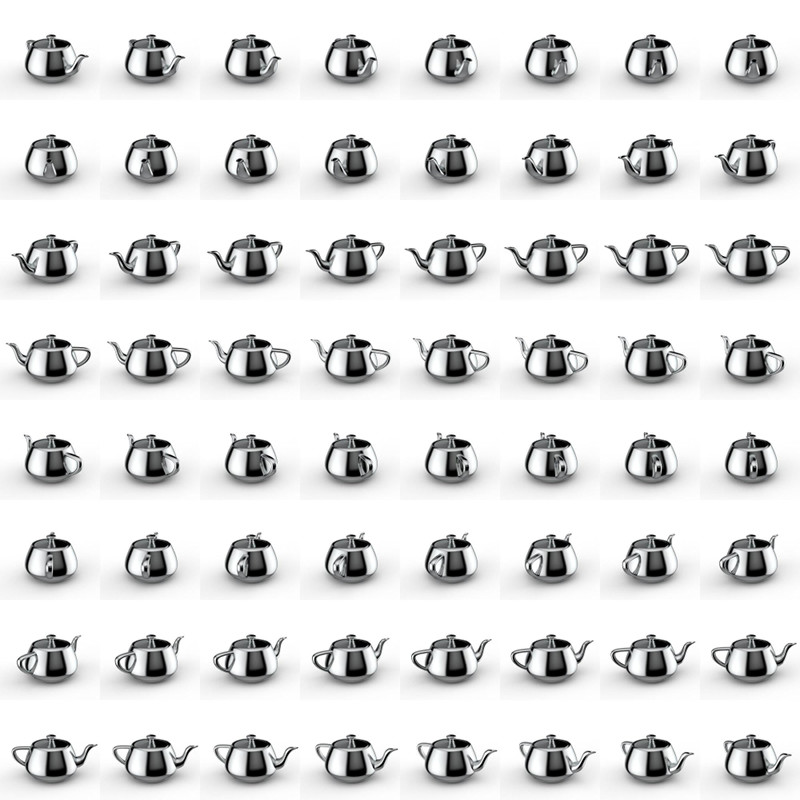# Create Turntable Animation with TitaniumGuest poster, Michael Gangolf, has been using Titanium since 2010, been a Titan since 2017 and Android lover since the beginning. You can follow him at @michaelgangolf or on Github at https://github.com/m1ga

Following the color animation tutorial, this post shows how to create turntable animation with Titanium. Turntable animation is a 360° animation in one axis:and is mostly used to show 3D objects from all sides. Since we cannot use a 3D engine, we will use a prerendered sprite sheet to create our animation. The sprite sheet contains all frames of the 360° animation and is stacked in a grid. The bigger you create the image, the higher the resolution will be; if you create more frames, you will have a smoother animation. In this example, we use a 8×8 grid with a 250x250px frame. So, the full image will be 2000x2000px.The Alloy setup is rather easy – a View with the whole image and one View around it that displays only one frame:

index.xml

``````<Alloy>
<Window>
<View id="frameView">
<View id="img"/>
</View>
</Window>
</Alloy>``````

index.tss

``````"#frameView" : {
width: 250,
height: 250,
touchEnabled: false
}
"#img" : {
width: 2000,
height: 2000,
backgroundImage: "/images/turntable.jpg",
touchEnabled: false,
top:0,
left:0
}``````

The whole logic is inside the controller where we use touchStart/touchMove to cycle through the frames. To do this, we have disabled `touchEnabled` on the two views and add two event listeners to our index window:

``````function onStart(e) {
}

function onMove(e) {
}

\$.index.open();``````

Inside onStart, we store the first x coordinate where the user clicks on the screen and calculate the distance to it every time touchmove is called:

``````var distX = 0;
var sX = 0;
var frame = 0;

function onStart(e) {
// set start value
sX = e.x;
}

function onMove(e) {
// calculate distance between start and current x
distX = (sX - e.x);
}

\$.index.open();``````

If we move 5px, we will change a frame and set the start point the the new x coordinate. When we reach frame 64, we have to go to frame 0 again and visa versa so we have an endless loop.

``````function onMove(e) {
// calculate distance between start and current x
distX = (sX - e.x);

// 5px buffer until we change a frame
if (distX < -5) { frame--; sX = Math.floor(e.x); } else if (distX > 5) {
frame++;
sX = Math.floor(e.x);
}

// check frame
if (frame >= 64) {
frame = 0;
} else if (frame < 0) {
frame = 63;
}
}``````

The last thing we need to do is calculate the top and left position of our frame. Frames 1 to 8 are at top=0 and left=0, 250, 500,…. Once we reach frame 9, we will start from left=0 again, but move top one row down (250px). In Javascript, we can do this simply with:

``````// frame to px
var x = Math.floor((frame * 250) % 2000);
var y = Math.floor((frame * 250) / 2000) * 250;

// change image
\$.img.left = -x;
\$.img.top = -y;``````

When you start your app and move your finger from one side to another, you will see a spinning object! The whole index.js looks like this:

``````var distX = 0;
var sX = 0;
var frame = 0;

function onStart(e) {
// set start value
sX = e.x;
}

function onMove(e) {
// calculate distance between start and current x
distX = (sX - e.x);

// 5px buffer until we change a frame
if (distX < -5) { frame--; sX = Math.floor(e.x); } else if (distX > 5) {
frame++;
sX = Math.floor(e.x);
}

// check frame
if (frame >= 64) {
frame = 0;
} else if (frame < 0) {
frame = 63;
}

// frame to px
var x = Math.floor((frame * 250) % 2000);
var y = Math.floor((frame * 250) / 2000) * 250;

// change image
\$.img.left = -x;
\$.img.top = -y;
}